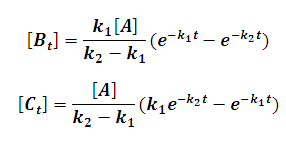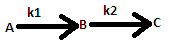# Product Yield of Sequential Reaction Calculator

This Calctown Calculator calculates the product yield of a sequential reaction.

mol/L
s-1
s-1
s

#### Result

mol/L
mol/Lwhere

Bt = concentration of B at time t

A = concentration of A initially

C = concentration of C at time t

k1 = reaction rate for 1st reaction

k2 = reaction rate for 2nd reaction

t = time